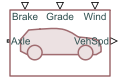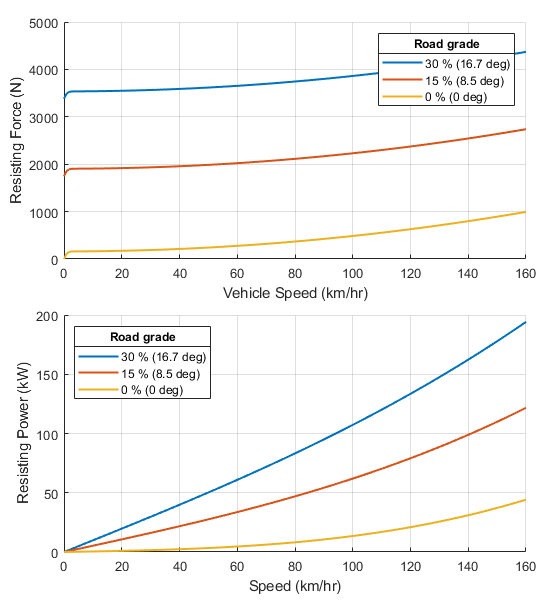# Longitudinal Vehicle

Longitudinal motion abstract vehicle with ideal brakes and tires

Since R2021b

•Libraries:
Simscape / Driveline / Tires & Vehicles

## Description

The Longitudinal Vehicle block represents an abstract vehicle confined to longitudinal motion. You can parameterize an arbitrary vehicle or choose from predefined parameterizations. The block includes optional non-slipping tires and ideal brakes.

You can use this block as a simpler alternative to the Vehicle Body block. The Longitudinal Vehicle block requires less information to parameterize and is more suitable for a nascent design.

The block equations use these variables:

• m is the Vehicle mass parameter.

• vx is the longitudinal velocity output at the VehSpd port.

• Fx is the sum of longitudinal forces.

• rtire is the radius of the tire and is equivalent to the Tire rolling radius parameter.

• Fdrive, Fbrake, and Fresist are the driving force of the vehicle, the braking force, and the vehicle resistance force, respectively. Fbrake and Fresist oppose the driving motion of the vehicle in the force balance equation.

• τaxle is the torque at the axle.

• FB is the brake force input from the Brake port.

• ωaxle is the rotational speed of the axle.

• ω1 is an arbitrary constant that adjusts the speed range in which the block suppresses Fbrake.

• Ftire is the tire rolling resistance.

• Fair is the drag force due to air resistance.

• θ is the road incline angle input from either the Angle, NG, or PG port.

• v1 is an arbitrary constant that adjusts the speed range in which the block suppresses Fair and Ftire.

You can access a visualization of the vehicle properties by clicking the vehicle properties hyperlink in the source code. The figure shows the default block properties, where the first plot is the resisting force as a function of vehicle speed for different road grades, and the second plot is the resisting power for a given speed at different road grades.### Parameterizations

For all settings of the Parameterization type parameter, the block calculates the equations of longitudinal vehicle speed as

`$m\frac{d{v}_{x}}{dt}=\sum {F}_{x},$`

where

`${v}_{x}={r}_{tire}{\omega }_{axle},$`

and

`$\sum {F}_{x}={F}_{drive}-{F}_{brake}-{F}_{resist}.$`

The block treats the drive direction as positive in the force balance equation. It solves this equation using these substitutions:

`$\sum {F}_{x}=\underset{{F}_{drive}}{\underbrace{\frac{{\tau }_{axle}}{{r}_{tire}}}}-\underset{{F}_{brake}}{\underbrace{{F}_{B}\mathrm{tanh}\left(\frac{{\omega }_{axle}}{{\omega }_{1}}\right)}}-\underset{{F}_{resist}}{\underbrace{\left\{\left({F}_{tire}\mathrm{cos}\theta +{F}_{air}\right)\mathrm{tanh}\left(\frac{{v}_{x}}{{v}_{1}}\right)+mg\mathrm{sin}\theta \right\}.}}$`

You can set Parameterization type to `Road-load` or ```Regular parameter set``` to simulate an arbitrary vehicle. You can also choose from three predefined parameterizations, `Typical small car`, `Typical medium-sized car`, and ```Typical large sports utility vehicle```.

Regular parameter set

When you set Parameterization type to ```Regular parameter set```, you can specify the Tire rolling coefficient, Air drag coefficient, and Vehicle frontal area parameters of the vehicle, which the block converts to road-load coefficients.

The block computes the tire rolling resistance as

`${F}_{tire}={C}_{R}mg,$`

where CR is the Tire rolling coefficient parameter.

The block computes the air resistance as

`${F}_{air}=\frac{1}{2}{C}_{D}{A}_{f}{\rho }_{a}{\left({v}_{x}+{v}_{w}\right)}^{2},$`

where CD is the Air drag coefficient parameter, Af is the Vehicle frontal area parameter in m2, and ρA is the density of dry air. The block assumes ρA = 1.184 kg/m3 at 1 atmosphere.

When you set Parameterization type to `Road-load`, the block applies the standard road-load formulation and computes the core physics as:

`${F}_{RL}=\underset{{F}_{tire}}{\underbrace{{A}_{RL}+{B}_{RL}{v}_{x}}}+\underset{{F}_{air}}{\underbrace{{C}_{RL}{v}_{x}^{2}}},$`

Note

Each road-load coefficient is specific to its associated units of vx. There are no standard units for these coefficients; however, some texts may assume certain units. Select the correct units when you parameterize these coefficients.

The block uses these coefficients to solve for the values in the core physics as

`${F}_{tire}={A}_{RL}+{B}_{RL}{v}_{x},$`

and

`${F}_{air}={C}_{RL}{\left({v}_{x}+{v}_{w}\right)}^{2}.$`

Here, vw is the wind speed from the Wind port.

Predefined Parameterizations

You can set Parameterization type to three predefined parameterizations: `Typical small car`, `Typical medium-sized car`, and `Typical large sports utility vehicle`. The values represent industry averages rather than specific vehicles. You can start with one of these predefined parameterizations and later adjust these values by setting Parameterization type to `Road-load` or ```Regular parameter set``` and using the pertinent information from the tables.

`Regular parameter set`

Parameterization typeVehicle massTire rolling radiusTire rolling coefficientDrag coefficientFrontal Area
`Typical small car`

`1100` `kg`

`0.3` `m`

`0.013`

`0.3`

0.9*1.65*1.45 = `2.153` `m^2`

```Typical medium-sized car```

`1800` `kg`

`0.3` `m`

`0.0136`

`0.31`

0.9*1.75*1.5 = `2.36` `m^2`

```Typical large sports utility vehicle```

`2600` `kg`

`0.4` `m`

`0.014`

`0.36`

0.9*1.88*1.85 = `3.13` `m^2`

Note that each predefined parameterization assumes B = 0 for the road-load method.

`Road-load`

Parameterization typeABC
`Typical small car`

`140.3` `N`

`0` `N/(m/s)`

`0.3824` `N*s^2/m^2`

```Typical medium-sized car```

`240.1` `N`

`0` `N/(m/s)`

`0.4336` `N*s^2/m^2`

```Typical large sports utility vehicle```

`357.1` `N`

`0` `N/(m/s)`

`0.6671` `N*s^2/m^2`

Where the Vehicle frontal area equation corresponds to 0.9·vehicle width·vehicle height.

### Variables

To set the priority and initial target values for the block variables prior to simulation, use the Initial Targets section in the block dialog box or Property Inspector. For more information, see Set Priority and Initial Target for Block Variables.

Nominal values provide a way to specify the expected magnitude of a variable in a model. Using system scaling based on nominal values increases the simulation robustness. Nominal values can come from different sources, one of which is the Nominal Values section in the block dialog box or Property Inspector. For more information, see Modify Nominal Values for a Block Variable.

## Ports

### Input

expand all

Physical signal input port associated with the brakes. The brake port ignores negative values.

#### Dependencies

To enable this port, set Show brake force inport to `On`.

Physical signal input port associated with the road incline, in radians. This value represents the angle of the road with respect to the horizontal surface at `0` and a 100% grade at `pi/4`.

#### Dependencies

To enable this port, set

• Show road slope inport to `On`

• Slope input type to `Incline angle`

Physical signal input port associated with the normalized road grade. A normalized road grade of `1` is equivalent to a π/4 radian angle.

#### Dependencies

To enable this port, set

• Show road slope inport to `On`

• Slope input type to `Normalized grade`

Physical signal input port associated with the percent road grade. A percent road grade of `100` is equivalent to a π/4 radian angle.

#### Dependencies

To enable this port, set

• Show road slope inport to `On`

• Slope input type to `Percent grade`

Physical signal input port associated with the longitudinal wind speed. This value is the longitudinal component of the wind speed, where positive values represent a headwind and negative values represent a tailwind.

#### Dependencies

To enable this port, set Show wind speed inport to `On`.

### Output

expand all

Physical signal output port associated with the longitudinal velocity of the vehicle.

Physical signal port associated with the ratio of the longitudinal vehicle acceleration, ax, to the gravitational acceleration, g.

#### Dependencies

To enable this port, set Show G outport to `On`.

### Conserving

expand all

Mechanical rotational conserving port associated with the axle connection to the wheel hub. Connect this port to a gear system such as a reduction gear.

## Parameters

expand all

### Vehicle

Option to parameterize the longitudinal vehicle. You can parameterize an arbitrary vehicle by choosing `Regular parameter set` or `Road-load`, or select one of three typical vehicle body specifications. Refer to Parameterizations for more information.

Effective mass of the longitudinal vehicle.

#### Dependencies

To enable this parameter, set Parameterization type to `Regular parameter set` or `Road-load`.

Distance from the wheel center to the outside surface of the tire while resisting the force of the vehicle due to gravity.

#### Dependencies

To enable this parameter, set Parameterization type to `Regular parameter set` or `Road-load`.

Dimensionless coefficient of the tire rolling resistance. The block uses this value to calculate Ftire as Ftire = CRmg. Here, the variable CR represents this parameter.

#### Dependencies

To enable this parameter, set Parameterization type to `Regular parameter set`.

Dimensionless coefficient of vehicular drag. The block uses this value to compute Fair as Fair = 1/2·CDAfρ(vx+vw)2, where CD represents this parameter.

#### Dependencies

To enable this parameter, set Parameterization type to `Regular parameter set`.

Effective area of the vehicle that succumbs to drag. You can use a low-fidelity heuristic formula to find this measurement during early design: Af = 0.9·height·width.

#### Dependencies

To enable this parameter, set Parameterization type to `Regular parameter set`.

#### Dependencies

To enable this parameter, set Parameterization type to `Road-load`.

#### Dependencies

To enable this parameter, set Parameterization type to `Road-load`.

#### Dependencies

To enable this parameter, set Parameterization type to `Road-load`.

Gravitational constant that acts on the mass of the vehicle as a function of the axle rotation. Here, vehicle weight = vehicle mass·gravitational acceleration. The block applies this weight to the tire to calculate the rolling resistance. The block also uses this value to determine the G-force multiple when you set Show G outport to `On`.

### Simulation

Minimum speed at which the block simulates vehicle motion. Below the absolute value of this threshold, the block smoothly transitions to zero.

Minimum rotational speed at which the block simulates axle rotation. Below the absolute value of this threshold, the block smoothly transitions to zero.

Option to display Brake port.

Option to enable a port to input data related to the road grade or slope. Setting this parameter to `On` enables the Slope input type parameter.

Option to input road slope. The options use these definitions:

• `Percent grade` — The block uses a percent grade scale where `0` is flat and `100` is equivalent to a slope of π/4.

• `Normalized grade` — The block uses a normalized grade scale where `0` is flat and `1` is equivalent to a slope of π/4.

• `Incline angle` — The block uses an angle of incline where `0` is flat and `pi/4` is equivalent to a slope of π/4.

#### Dependencies

To enable this parameter, set Show road slope inport to `On`.

Option to display the Wind port.

Option to display the G port. record G-force as a multiple of the Gravitational acceleration parameter.

 Eriksson, Lars, and Lars Nielsen. Modeling and Control of Engines and Drivelines: Eriksson/Modeling and Control of Engines and Drivelines. Chichester, UK: John Wiley & Sons, Ltd, 2014. https://doi.org/10.1002/9781118536186.

 Heywood, John B. Internal Combustion Engine Fundamentals. Second edition. New York: McGraw-Hill Education, 2018.

 Society of Automotive Engineers: Light Duty Vehicle Performance and Economy Measure Committee. “Chassis Dynamometer Simulation of Road Load Using Coastdown Techniques. SAE J2264” SAE International. 2014. https://doi.org/10.4271/J2264_201401.

 Society of Automotive Engineers: Light Duty Vehicle Performance and Economy Measure Committee. “Road Load Measurement and Dynamometer Simulation Using Coastdown Techniques. SAE J1263” SAE International. 2010. https://doi.org/10.4271/J1263_201003.